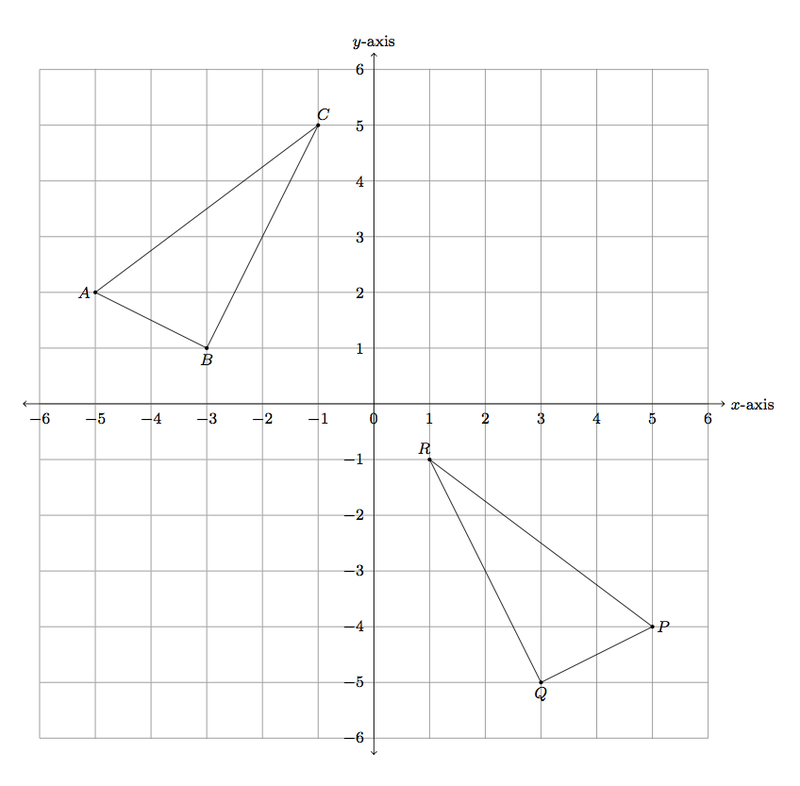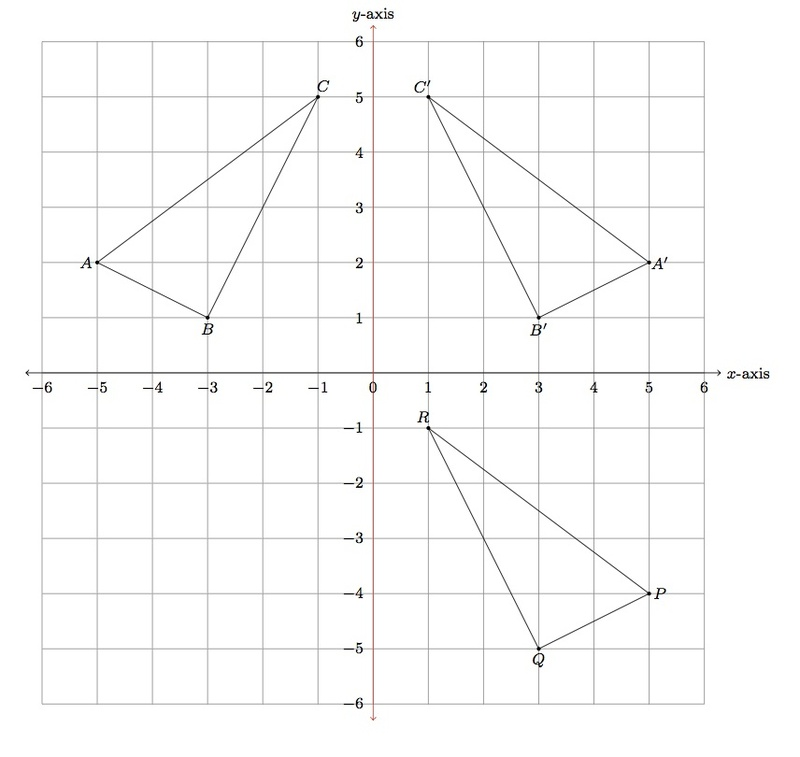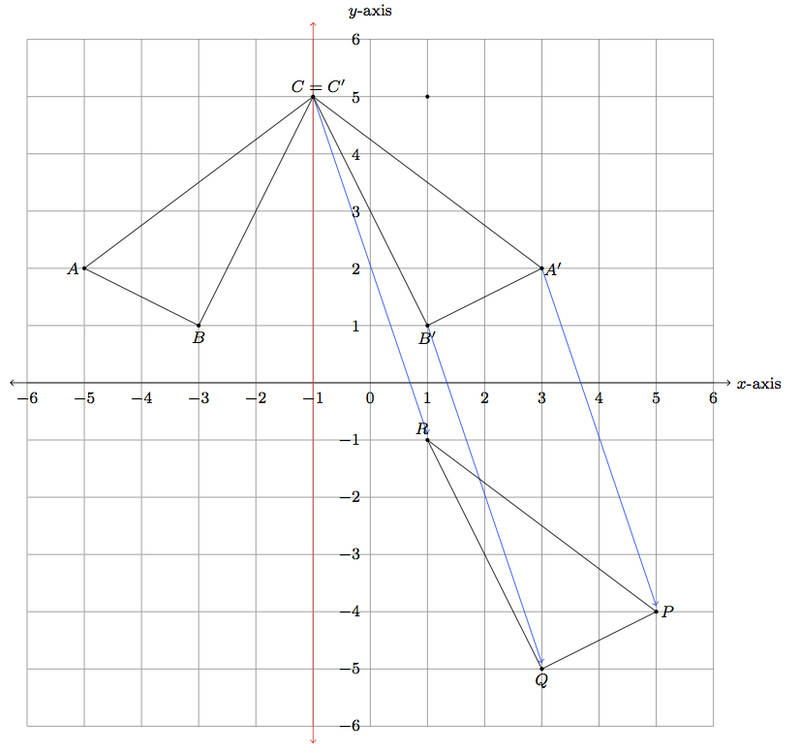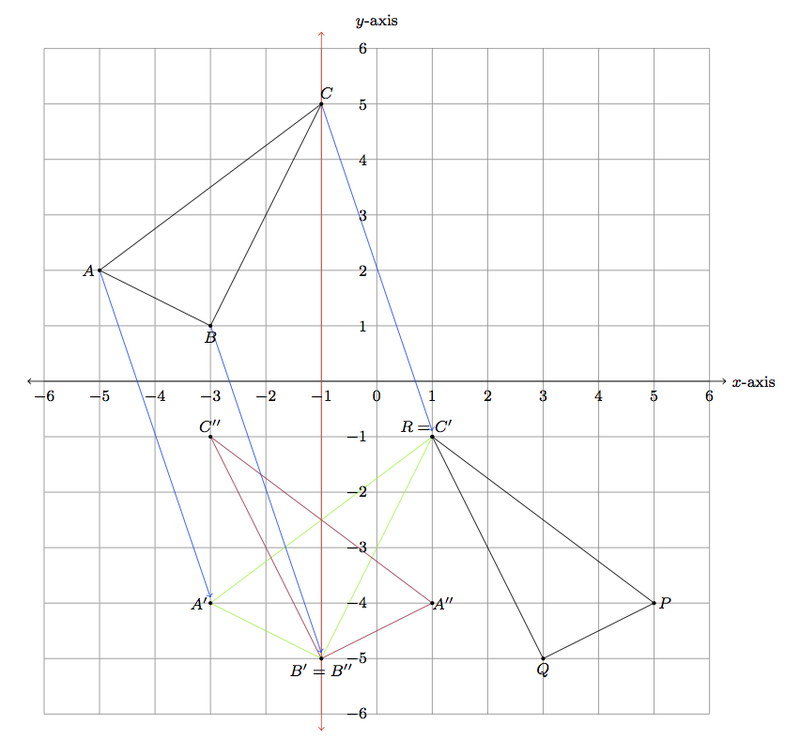# Triangle congruence with coordinates

Alignments to Content Standards: 8.G.A.2 8.G.A.3

Triangles $ABC$ and $PQR$ are shown below in the coordinate plane:1. Show that $\triangle ABC$ is congruent to $\triangle PQR$ with a reflection followed by a translation.
2. If you reverse the order of your reflection and translation in part (a) does it still map $\triangle ABC$ to $\triangle PQR$?
3. Find a second way, different from your work in part (a), to map $\triangle ABC$ to $\triangle PQR$ using translations, rotations, and/or reflections.

## IM Commentary

This task gives students a chance to explore several issues relating to rigid motions of the plane and triangle congruence. First, part (a) gives students an opportunity to describe rigid motions in the coordinate plane to exhibit a congruence between two explicit triangles. There are two reasons to ask students to first find a reflection followed by a translation that will show the congruence. One is that there are multiple combinations of reflections followed by translations that will work, giving students a chance to see that there is more than one way to do this. The other is to set up the conversation that can result after students do part (b).

Part (b) looks more deeply into the different types of rigid motions (translations, rotations, and reflections) examining whether or not it matters in which order these transformations are applied: this depends very much on the specific operations performed as is shown in the solution with some examples. If the students do not produce an example where the order of the reflection and translation influences the end result, the teacher should be prepared to discuss an example similar to the one provided in part (b) of the solution.

Finally, part (c) gives students a chance to think creatively about showing the congruence between the two triangles without having the steps prescribed. Encourage students to find several different ways if they find another one immediately.

This task is appropriate for instruction. It illustrates both 8.G.A.2 because, as an instructional task, it can help students build up their understanding of the relationship between rigid motions and congruence. It also addresses 8.G.3.A: while the students have not been prompted to explicitly describe the transformations in terms of coordinates, they will necessarily use them at least implicitly. If it is appropriate, the teacher can give extra instructions indicating that students need to describe the transformations explicitly in terms of coordinates; alternatively, the teacher can use this task could lead into a discussion about how to describe each of the transformations using coordinates. Part (b) is intended to provide extra depth of understanding to rigid motions and congruence. It provides students with an important example of a context where order of operations matters (in this case the operations being transformations of the plane).

## Solution

1. Below the $y$-axis is shaded red and triangle $ABC$ is reflected over the $y$-axis. The image of this reflection is triangle $A^\prime B^\prime C^\prime$. Reflecting about the $y$-axis leaves the $y$-coordinate of each point the same and switches the sign of the $x$-coordinate.So, for example, $A = (-5,2)$ so $A^\prime = (5,2)$. We can now see that translating triangle $A^\prime B^\prime C^\prime$ down by $6$ units puts it on top of triangle $PQR$:To find the coordinates after applying this translation, the $x$-coordinate stays the same and we subtract 6 from the $y$-coordinate of each point.
2. The answer here will depend on which reflection and translation have been chosen in part (a). For the reflection and translation chosen above, we reverse the order by first translating $\triangle ABC$ by $6$ units downward and then reflecting over the $y$-axis. Below, the translated triangle is triangle $A^\prime B^\prime C^\prime$ and its reflection over the $y$-axis is $\triangle PQR$:Below is a different reflection through the vertical line through vertex $A$, which can be followed by the translation indicated by the blue arrows to show the congruence of $\triangle ABC$ with $\triangle PQR$:Unlike in the previous case, if we perform the translation first, giving the green triangle $A^\prime B^\prime C^\prime$, and then the reflection, giving the purple triangle $A^{\prime \prime} B^{\prime \prime} C^{\prime \prime}$, this does not produce the triangle $PQR$. So in this case, performing the translation and reflection in a different order produces a different outcome.3. One way to show the triangle congruence would be to align one vertex at a time. Graphically this is shown below:First a translation is used to move $C$ to $R$ with the new triangle shown in green. If $B^\prime$ is the image of $B$ under this translation, then a rotation, by the directed angle indicated in purple, moves $B^\prime$ to $Q$: the triangle after this transformation is indicated in blue, sharing one side with triangle $PQR$. If $A''$ is the image of $A$ after the translation and rotation, then a reflection across $\overline{QR}$ moves $A''$ to $P$.